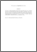# Prime gamma-near-rings with (σ, τ)-derivations

## Citation

Rakhimov, Isamiddin Sattarovich and Dey, Kalyan Kumar and Paul, Akhil Chandra (2013) Prime gamma-near-rings with (σ, τ)-derivations. International Journal of Pure and Applied Mathematics, 82 (5). pp. 669-681. ISSN 1311-8080; ESSN: 1314-3395

## Abstract

Let N be a 2 torsion free prime Γ-near-ring with center Z(N) and let d be a nontrivial derivation on N such that d(N) ⊆ Z(N). Then we prove that N is commutative. Also we prove that if d be a nonzero (σ,τ)-derivation on N such that d(N) commutes with an element aofN then ether d is trivial or a is in Z(N). Finally if d1 be a nonzero (σ,τ)-derivation and d2 be a nonzero derivation on N such that d1τ = τ d1, d1σ = σd1, d2τ = τ d2, d2σ = σd2 with d1(N)Γσ(d2(N)) = τ(d2(N))Γd1(N) then N is a commutative Γ-ring.Preview
PDF (Abstract)
Prime gamma.pdfView Item